F

i

v

e

-

gr

ea

t

### 问题 1459: [蓝桥杯][2013年第四届真题]高僧斗法

``````1  5  9
``````

``````1 4
``````Nim 的做法 就是 将 2 3 4 都转化为2进制再 异或 得出结果，如果结果是非0 那么先手必定赢 如果结果为0 那么先手必输（前提，玩游戏的都想赢 且都很聪明）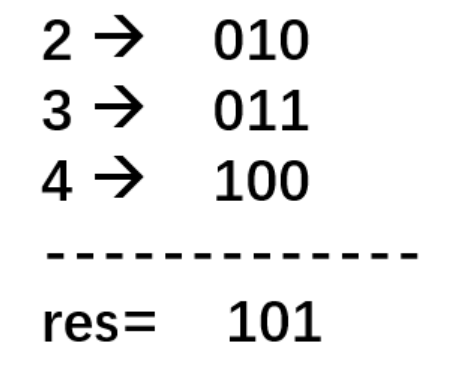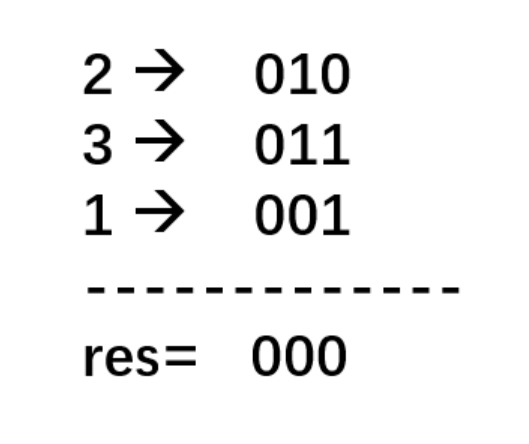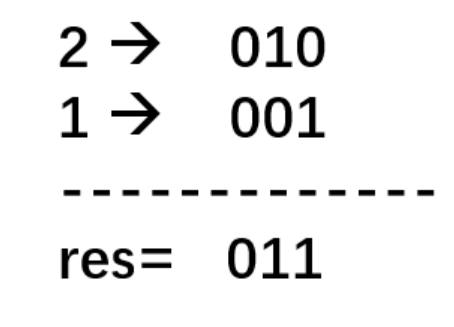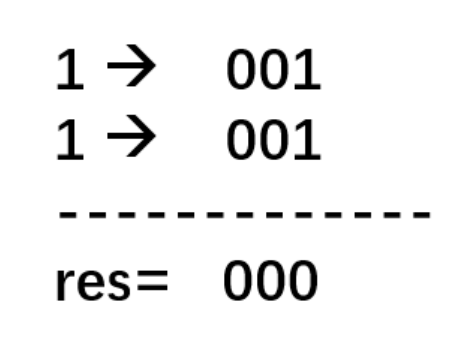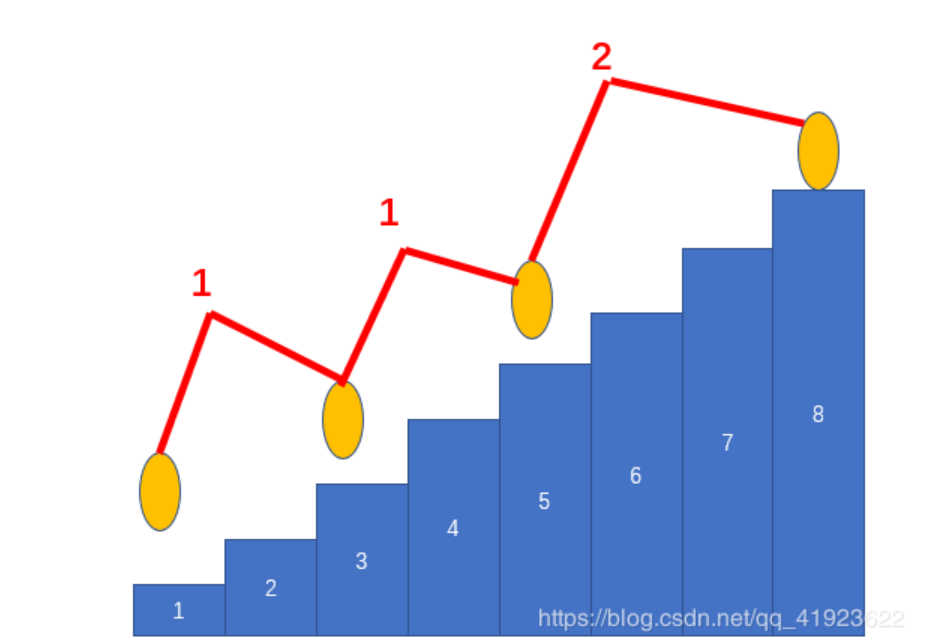##AC代码

``````#include<iostream>
#define N 102
using namespace std;
int main(){
int a[N],b[N];
int n = 0,i,j,k,sum = 0;
while(cin>>a[n])n++;//存储又有多少个小和尚
for(i=1; i<n; i++)b[i-1] = a[i] - a[i-1] - 1;// 进行Nim博弈的转换
for(i=0; i<n-1; i+=2) sum ^= b[i];//进行异或
if(sum==0)cout<<-1<<endl;//若开始局面为0 则必输
else//若非0 则必赢，因此 需要找到第一步 将局面变为0 的步骤
{
for(i=0; i<n-1; ++i)//枚举移动第i堆  使得剩下的局面异或等于0，
for(j=1; a[i]+j<a[i+1]; ++j) {//枚举可以移动的步数  保证 前项移动j 步后 不会超过后项
b[i] -= j;//拿走 j个 ，这里代表 前一个向上移动j步
if(i!=0)b[i-1] += j;//它的后一堆b[i]向取走了j个，那莫前一堆 b[i-1] 则要增加j个 第一堆除外
sum = 0;
for(k=0; k<n-1; k+=2) sum ^= b[k];//重新计算局面，
if(sum==0) {cout<<a[i]<<" "<<a[i]+j<<endl; break;}//若变成0  则后手必败，先手必赢。跳出即可；
b[i] += j;//回溯 这不是必赢的操作
if(i!=0) b[i-1] -= j;
}
}
return 0;
}
``````